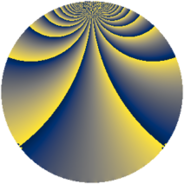# Properties

 Label 770.2.bdLevel $770$ Weight $2$ Character orbit 770.bd Rep. character $\chi_{770}(263,\cdot)$ Character field $\Q(\zeta_{12})$ Dimension $192$ Newform subspaces $1$ Sturm bound $288$ Trace bound $0$

# Related objects

## Defining parameters

 Level: $$N$$ $$=$$ $$770 = 2 \cdot 5 \cdot 7 \cdot 11$$ Weight: $$k$$ $$=$$ $$2$$ Character orbit: $$[\chi]$$ $$=$$ 770.bd (of order $$12$$ and degree $$4$$) Character conductor: $$\operatorname{cond}(\chi)$$ $$=$$ $$385$$ Character field: $$\Q(\zeta_{12})$$ Newform subspaces: $$1$$ Sturm bound: $$288$$ Trace bound: $$0$$

## Dimensions

The following table gives the dimensions of various subspaces of $$M_{2}(770, [\chi])$$.

Total New Old
Modular forms 608 192 416
Cusp forms 544 192 352
Eisenstein series 64 0 64

## Trace form

 $$192q + 8q^{5} + O(q^{10})$$ $$192q + 8q^{5} + 4q^{11} + 16q^{15} + 96q^{16} + 24q^{22} - 8q^{23} + 24q^{25} - 8q^{26} - 16q^{33} - 160q^{36} - 40q^{37} - 40q^{42} + 32q^{45} - 40q^{47} + 40q^{53} + 8q^{55} + 16q^{56} + 32q^{58} - 48q^{67} - 72q^{70} + 32q^{71} - 48q^{75} - 72q^{77} - 8q^{80} + 64q^{81} + 16q^{82} - 12q^{88} - 112q^{91} - 16q^{92} + 80q^{93} - 64q^{97} + O(q^{100})$$

## Decomposition of $$S_{2}^{\mathrm{new}}(770, [\chi])$$ into newform subspaces

Label Dim. $$A$$ Field CM Traces $q$-expansion
$$a_2$$ $$a_3$$ $$a_5$$ $$a_7$$
770.2.bd.a $$192$$ $$6.148$$ None $$0$$ $$0$$ $$8$$ $$0$$

## Decomposition of $$S_{2}^{\mathrm{old}}(770, [\chi])$$ into lower level spaces

$$S_{2}^{\mathrm{old}}(770, [\chi]) \cong$$ $$S_{2}^{\mathrm{new}}(385, [\chi])$$$$^{\oplus 2}$$